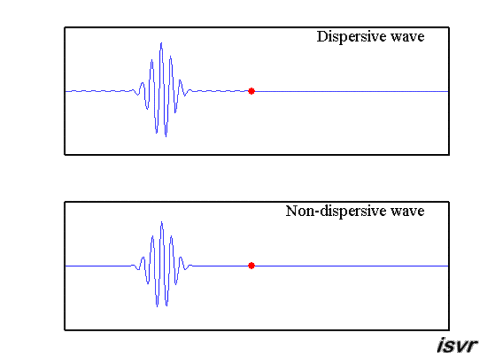## Dispersive waves

In sound waves, and for waves on strings, the wave velocity is the same at all frequencies. Flexural waves can also propagate in bars and plates but here the wave velocity varies with frequency. Higher-frequency waves travel faster than lower-frequency waves. These differences in speed cause spreading or dispersion of wave packets, as shown in the movie below.#### Group velocity and phase velocity

The group velocity is the speed of the wavepacket and the phase velocity is the speed of the individual waves. The following movies show wave packets with various combinations of phase and group velocities.#### Phase velocity = Group Velocity

The entire waveform – the component waves and their envelope – moves as one. This is an example of a non-dispersive wave.

#### Phase velocity = -Group Velocity

The envelope moves in the opposite direction of the component waves.

#### Phase velocity > Group Velocity

The component waves move more quickly than the envelope.

#### Phase velocity < Group Velocity

The component waves move more slowly than the envelope.

#### Group Velocity = 0

The envelope is stationary while the component waves move through it.

#### Phase velocity = 0

Now only the envelope moves over stationary component waves.